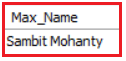# MySQL MIN and MAX Function

## MySQL MIN and MAX Function with Examples

In this article, I am going to discuss MySQL MIN and MAX Function with Examples. Please read our previous article where we discussed MySQL SUM Function with Examples.

##### Understanding MIN and MAX Functions in MySQL:

We are going to use the following Employee table to understand the MySQL MIN and MAX function with Examples.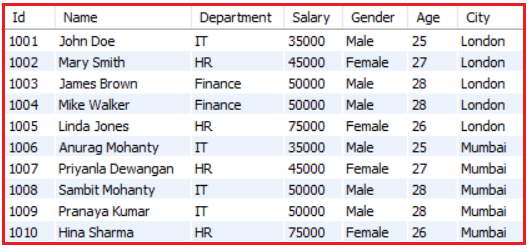Please use the following SQL Script to create and populate the Employee table with the required sample data.

```CREATE DATABASE Company;
USE Company;

CREATE TABLE Employee (
Id INT PRIMARY KEY,
Name VARCHAR(45) NOT NULL,
Department VARCHAR(45) NOT NULL,
Salary FLOAT NOT NULL,
Gender VARCHAR(45) NOT NULL,
Age INT NOT NULL,
City VARCHAR(45) NOT NULL
);

INSERT INTO Employee VALUES (1001, 'John Doe', 'IT', 35000, 'Male', 25, 'London');
INSERT INTO Employee VALUES (1002, 'Mary Smith', 'HR', 45000, 'Female', 27, 'London');
INSERT INTO Employee VALUES (1003, 'James Brown', 'Finance', 50000, 'Male', 28, 'London');
INSERT INTO Employee VALUES (1004, 'Mike Walker', 'Finance', 50000, 'Male', 28, 'London');
INSERT INTO Employee VALUES (1005, 'Linda Jones', 'HR', 75000, 'Female', 26, 'London');
INSERT INTO Employee VALUES (1006, 'Anurag Mohanty', 'IT', 35000, 'Male', 25, 'Mumbai');
INSERT INTO Employee VALUES (1007, 'Priyanla Dewangan', 'HR', 45000, 'Female', 27, 'Mumbai');
INSERT INTO Employee VALUES (1008, 'Sambit Mohanty', 'IT', 50000, 'Male', 28, 'Mumbai');
INSERT INTO Employee VALUES (1009, 'Pranaya Kumar', 'IT', 50000, 'Male', 28, 'Mumbai');
INSERT INTO Employee VALUES (1010, 'Hina Sharma', 'HR', 75000, 'Female', 26, 'Mumbai');
```
##### MySQL MIN() Function

The MIN or MINIMUM function returns the smallest value of the given column. The MIN function works on numeric columns as well as string columns. The following SQL statement showing how to use of MIN function in MySQL.

SELECT MIN(columnname) FROM tablename;

##### Example: MySQL MIN() Function with Numeric Data Type

Our requirement is to find the MINIMUM age from the Employees table. To do so, we need to pass the Age column to the MIN function as shown in the below SQL query.

SELECT MIN(Age) AS Min_AGE FROM Employee;

When you execute the above SELECT Statement, you will get the following output. Here, we get the smallest value in the age column of the employee table i.e. 25.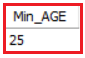##### Example: MIN function with GROUP by Clause in MySQL

Now we need to find the minimum salary in each department. To find the same, we need to pass the salary column to the MIN function as well as we need to group the employees by the department by using the GROUP by clause as shown in the below query.

```SELECT Department, MIN(Salary) AS Min_Salary
FROM Employee
GROUP BY Department;```

When you execute the above SELECT query, you will get the following output.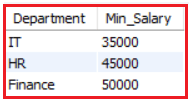##### Example: MIN Function with WHERE Clause in MySQL

Now we need to find the minimum salary of Male Employees. To do so, we need to pass the Salary column to the MIN function and filter the Male employees by using the WHERE clause as shown in the below query.

```SELECT MIN(Salary) AS Min_Salary
FROM Employee
WHERE Gender = 'Male';```

When you execute the above SELECT query, you will get the following output.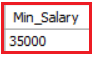##### Example: MIN Function with String Values in MySQL

We can also use the MIN function on the string column like the below example.

SELECT MIN(Name) AS Min_Name FROM Employee;

When you execute the above SQL query, you will get the following output. Here, we get the minimum value that is alphabetically the smallest value in the name column. For string column values, the MIN function arranges the values alphabetically and gets the first ascending value from the column.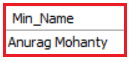##### MySQL MAX() Function

The MAX or MAXIMUM function returns the largest value of the given column. The MAX function works on numeric columns as well as string columns. The following SQL statement showing how to use of MAX function in MySQL.

SELECT MAX(columnname) FROM tablename;

##### Example: MAX Function with Numeric Data Type in MySQL

Our requirement is to find the MAXIMUM age from the Employees table. To do so, we need to pass the Age column to the MAX function as shown in the below SQL query.

SELECT MAX(Age) AS Max_AGE FROM Employee;

When you execute the above SELECT Statement, you will get the following output. Here, we get the highest value in the age column of the employee table i.e. 28.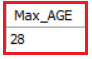##### Example: MAX Function with GROUP by Clause in MySQL

Now we need to find the highest salary in each department. To find the same, we need to pass the salary column to the MAX function as well as we need to group the employees by the department by using the GROUP by clause as shown in the below query.

```SELECT Department, MAX(Salary) AS Max_Salary
FROM Employee
GROUP BY Department;```

When you execute the above SELECT query, you will get the following output.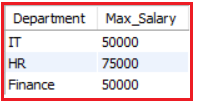##### Example: MAX Function with WHERE Clause in MySQL

Now we need to find the highest salary of Male Employees. To do so, we need to pass the Salary column to the MAX function and filter the Male employees by using the WHERE clause as shown in the below query.

```SELECT MAX(Salary) AS Max_Salary
FROM Employee
WHERE Gender = 'Male';```

When you execute the above SELECT query, you will get the following output.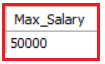##### Example: MAX Function with String Values in MySQL

We can also use the MySQL MAX function on the string column like the below example.

SELECT MAX(Name) AS Max_Name FROM Employee;

When you execute the above SQL query, you will get the following output. Here, we get the highest value that is alphabetically the highest value in the name column. For string column values, the MAX function arranges the values alphabetically and gets the first descending value from the column.